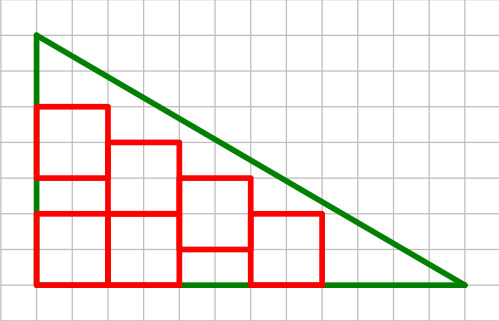# Programming contests

## DEIK Regionális Programozó Csapatverseny, középiskolai kategória, 2022. december 4.

December 4, 2022 10:00 AM – December 4, 2022 3:15 PM

# squaresWhat is the maximal number of squares with side lengths of `K` that can be packed - without overlaps - into a right triangle whose legs are of lengths `A` and `B`? For each square one of its side must be parallel with one of the triangles leg!

## Example

In the figure above one can see the solution (`M=6`) in the case of `K=2,A=7,B=12`.

## Input Specification

``````
T
K1 A1 B1
...
KT AT BT
```
```

The first line is the number cases to be solved. Then `T` lines follow, each of which with three space separated numbers `Ki,Ai,Bi`.

``````
1 ≤ T ≤ 100
1 ≤ Ki ≤ 100
1 ≤ Ai,Bi ≤ 104 (i=1...T)
```
```

## Output Specification

``````
M1
...
MT
```
```

`T` lines with the required values of `Mi (i=1...T)`.

## Sample Input 1

1. `1`
2. `2 7 12`

1. `6`

## Sample Input 2

1. `4`
2. `2 10 10`
3. `2 3 3`
4. `17 1717 17171`
5. `71 7171 71717`
1. `10`
2. `0`
3. `50500`
4. `50500`